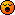# Mathematics! Help!

#### Gammawolf

Associate
Hey there, just a question for all you maths geniuses out there! Say I have the equation:

B = g^b mod p

I know all the values except for b, and p is a prime number which is also known. How do I go about finding b, I can't for the life of me figure this out because of the mod operator! Reversing it just confuses meDoes anyone know where I can get started to solve this one? If it helps its in relation to Diffie-Hellman key exchange methods#### Amleto

Soldato
you have one equation in two unknowns... there is not a single solution.

#### Gammawolf

Associate
OP
Where is the second unknown? Are you referring to the g^b before the modulus operator is applied?

#### Amleto

Soldato
sorry, just mis-read first sentence after equation

Last edited:

#### Amleto

Soldato
I can't remember modular algebra, but don't you get a 2nd unknown though this operation anyway:

B = g^b mod p
multiply by p =>

Np + B = g^b

for some integer N

#### PanMaster

Associate
B = (g^b)%p

b = log(B + N.p)/log(g)

#### Amleto

Soldato
Pan, I didn't think it needed saying#### Gammawolf

Associate
OP
So basically my cryptography lecturer is having one on with me? It is the last one of a set of 30 questions so its not unlikely that he's just set one that cannot be feasibly done